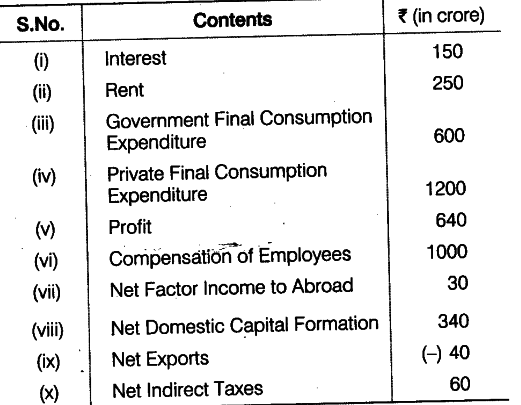# Calculate National Income by

Calculate National Income by (a) Income method and (b) Expenditure method from the following data:(a) By income method,
National Income (NN\${{P}{FC}}\$) = Compensation of Employees + Rent + Interest + Profits - Net Factor Income to Abroad =1000+250+150+640 -30 =Rs. 2010 crore
(b) By expenditure method
National Income (NN\${{P}
{FC}}\$)
= Private Final Consumption Expenditure + Government Final Consumption Expenditure + Net Domestic Capital Formation+Net Export - Net Indirect Taxes - Net Factor Income to Abroad
= 1200 +600 + 340 + (-40)-60 - 30 = Rs. 2010 crore